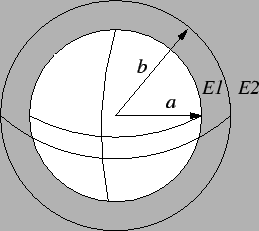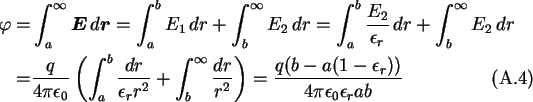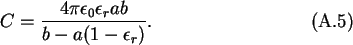Next: A.3 Capacitance of Two Up: A Capacitance Calculations Previous: A.1 Self Capacitance of

# A.2 Self Capacitance of a Sphere with a Dielectric ShellThe capacitance of a conducting sphere with a dielectric shell, as shown in Fig. A.1, is calculated using the boundary conditionThe potential of the sphere is calculated by a path integral from the surface of the sphere to infinity over the electric field.And hence the capacitance of a conducting sphere with a dielectric shell isChristoph Wasshuber